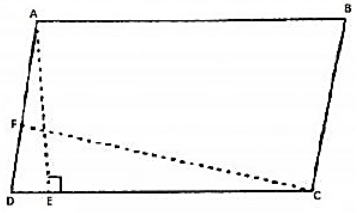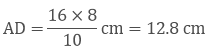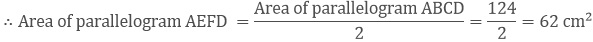×#### Thank you for registering.

One of our academic counsellors will contact you within 1 working day.

Click to Chat

1800-1023-196

+91-120-4616500

CART 0

• 0

MY CART (5)

Use Coupon: CART20 and get 20% off on all online Study Material

ITEM
DETAILS
MRP
DISCOUNT
FINAL PRICE
Total Price: Rs.

There are no items in this cart.
Continue Shopping• Complete JEE Main/Advanced Course and Test Series
• OFFERED PRICE: Rs. 15,900
• View Details

```Chapter 15: Areas of Parallelograms And Triangles Exercise – 15.2

Question: 1

If figure, ABCD is a parallelogram, AE ⊥ DC and CF ⊥ AD. If AB = 16 cm, AE = 8 cm, and CF = 10 cm, Find AD.Solution:

Given that,

In parallelogram ABCD, CD = AB = 16 cm         [∵ Opposite side of a parallelogram are equal]

We know that,

Area of parallelogram = Base × Corresponding altitude

Area of parallelogram ABCD = CD × AE = AD × CF

16 cm × cm = AD × 10 cmThus, The length of AD is 12.8 cm.

Question: 2

In Q1, if AD = 6 cm, CF = 10 cm, and AE = 8 cm, Find AB.

Solution:

We know that,

Area of a parallelogram ABCD = AD × CF ⋅⋅⋅⋅⋅⋅ (1)

Again area of parallelogram ABCD = CD × AE⋅⋅⋅⋅⋅⋅ (2)

Compare equation (1) and equation (2)

AD × CF = CD × AE

⇒ 6 × 10 = D × 8

⇒ D = 60/8 = 7.5 cm

∴ AB = DC = 7.5 cm     [∵ Opposite side of a parallelogram are equal]

Question: 3

Let ABCD be a parallelogram of area 124 cm2. If E and F are the mid-points of sides AB and CD respectively, then find the area of parallelogram AEFD.

Solution:

Given,

Area of a parallelogram ABCD = 124 cm2

Construction: Draw AP⊥DC

Proof:-

Area of a parallelogram AFED = DF × AP ⋅⋅⋅⋅⋅⋅⋅⋅ (1)

And area of parallelogram EBCF = FC × AP⋅⋅⋅⋅⋅⋅⋅⋅ (2)

And DF = FC  ⋅⋅⋅⋅⋅ (3)      [F is the midpoint of DC]

Compare equation (1), (2) and (3)

Area of parallelogram AEFD = Area of parallelogram EBCFQuestion: 4

If ABCD is a parallelogram, then prove that

Ar (ΔABD) = Ar(ΔBCD) = Ar(ΔABC) = Ar(ΔACD) = (1/2) Ar (// gm ABCD).

Solution:

Given:-

ABCD is a parallelogram,

To prove: - Ar (ΔABD) = Ar(ΔBCD) = Ar(ΔABC) = Ar(ΔACD) = (1/2) Ar (//gm ABCD).

Proof:- We know that diagonal of a parallelogram divides it into two equilaterals .

Since, AC is the diagonal.

Then, Ar (ΔABC) = Ar(ΔACD) = (1/2) Ar(// gm ABCD)   ⋅⋅⋅⋅ (1)

Since, BD is the diagonal.

Then,  Ar(ΔABD) = Ar(ΔBCD) = (1/2) Ar(// gm ABCD)   ⋅⋅⋅⋅⋅ (2)

Compare equation (1) and (2)

∴  Ar(ΔABC) = Ar(ΔACD) = Ar(ΔABD) = Ar(ΔBCD) = (1/2) Ar(// gm ABCD)..
```### Course Features

• 728 Video Lectures
• Revision Notes
• Previous Year Papers
• Mind Map
• Study Planner
• NCERT Solutions
• Discussion Forum
• Test paper with Video Solution